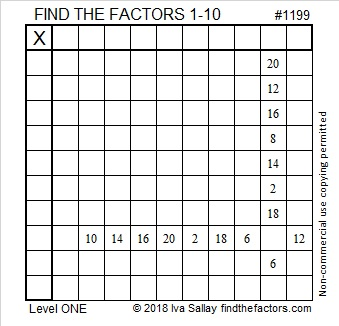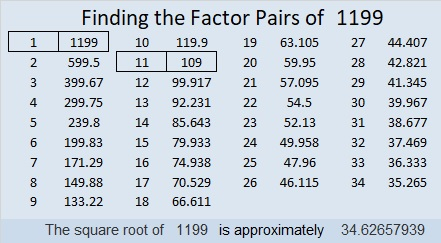# 1199 and Level 1

Here’s a puzzle that even someone just learning to multiply and divide can solve. That means you can solve it, too!Print the puzzles or type the solution in this excel file: 10-factors-1199-1210

Here are some facts about the number 1199:

• 1199 is a composite number.
• Prime factorization: 1199 = 11 × 109
• The exponents in the prime factorization are 1 and 1. Adding one to each and multiplying we get (1 + 1)(1 + 1) = 2 × 2 = 4. Therefore 1199 has exactly 4 factors.
• Factors of 1199: 1, 11, 109, 1199
• Factor pairs: 1199 = 1 × 1199 or 11 × 109
• 1199 has no square factors that allow its square root to be simplified. √1199 ≈ 34.626581199 is the sum of the fifteen prime numbers from 47 to 109. That last one just happens to be one of its prime factors, too!

1199 is the hypotenuse of a Pythagorean triple:
660-1001-1199 which is 11 times (60-91-109)

1199 looks cool in base 10, and it’s palindrome
2F2 in BASE 21 (F is 15 base 10)

This site uses Akismet to reduce spam. Learn how your comment data is processed.